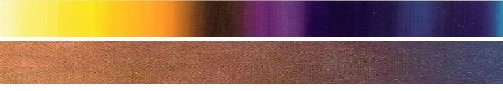# Basics of Radiation Power Transfer

When an object (more correctly a surface) is at a temperature above 0K (Zero Kelvin) it emits a spectrum of radiation, called the electromagnetic (em) radiation. The object can be thought to have a natural glow with an intensity and type of glow which depends on the temperature of the surface. Such emitted radiation can be thought to be wave-like with many frequencies  i.e. display a spectrum of wavelengths - same as velocity/frequency. The velocity of em radiation in vacuum is fixed.  The radiation can also be alternatively thought of comprising of massless photons with a probability distribution of energy that are emitted that depends on T, the temperature in Kelvin (more correctly, depends on kT where k is the Boltzmann constant).  Such a photon based explanation of radiation is the more accepted theory of light/electromagnetic radiation, as it is the quantum-mechanical description of light.  According to our present understanding, the electromagnetic field itself is produced by photons, which in turn result from a local gauge symmetry and the laws of quantum field theory.  However for heat transfer calculations, the wave particle duality is not of critical importance because the experimental results follow the Stefan-Boltzmann and Planck's radiation laws discussed below.

The maximum rate of radiation emitted (Power) by a surface with an area A, integrated over all wavelengths of the radiation, is given by the Stefan-Boltzmann Law which is written as: Radiated Power Intensity, P (W) = e.s.A.Te^4  where e is the emissivity, Te is the temperature in Kelvin, of the emitting surface and s is the Stefan-Boltzmann Constant = 5.6703 x 10-8 W/m2K4The emissivity (e) is a relatively complex variable that encompasses several laws of physics. The emissivity depends on temperature, wavelength and the angle of emission. It is the ratio of power radiated by a real material to the power radiated by a black-body at the same temperature.   For a black-body e = 1.  The emissivity e ranges from 0-1 for solid materials.  Radiation can exert presssure - a simple way of thinking about this is that the rest mass of photons is zero but when they travel at the velocity of light - their effective mass is non-zero, thus they have momentum and can exert force when encountering an obstruction.  It is the effective mass from E=m.c^2 that infers a vector momentum, which when changed gives rise to a vector -force.  However, do note that 1 J of energy is ~.11 x10^-16 Kg for em radiation.  Compare with 9.1 x 10^-31Kg as the mass of an electron at rest. So the photons can exert a punch on electrons as described by the Ohio born AH Compton (1892-1962) for inelastic scattering. The plot of radiation power per unit area as a function of temperature (the Stefan-Boltzmann law) is shown below.  A table that illustrates how steeply the total radiation power increases with the surface temperature of the emission surface is also shown.  Further-on below, a discussion of the spectrum of wavelengths or the probability of a certain frequency range of photons emitted is presented (i.e. the Planck's radiation law).Comparing typical Gas Burners to Electric GlowPanels™ ?

Compare the Power and Temperature

Power Rating Conversion

90,000 BTU/hour = 26.4 kiloWatts (kW)

The radiation intensity increases with temperature

 Material that is Combusted/ Burned Typical Flame Temperature Average* (approximate) Natural Gas (Methane) 900~1500°C (1652-2732°F) Charcoal Fire 750~1200°C (1382-2192°F) Typical Candle Flame 1100°C (2012°F) No combustion. MHI GlowPanel™ Up to 1900°C (3452°F) (Several models 1400°C - 1900°C )

* Source:  https://en.wikipedia.org/wiki/Flame. Note that the average temperature is not the peak

temperature which could be higher.  MHI patented materials are considered in the comparison.

Glow panels offer non-turbulent heating.

An important law called Kirchhoff's law states that for a material in thermal equilibrium the emissivity el, at any given wavelength and the absorptivity al at the same wavelength are equal (at a fixed surface temperature).  A black-body is a perfect absorber as well as a perfect emitter. When radiation encounters an object, it may be absorbed, reflected and/or refracted/transmitted.  For heat (infra-red radiation) the transmission and refraction may only be through a few atomic layers where the energy is "absorbed".  One may therefore ignore transmission through more than a few atomic layers for such thermal (heat) radiation ( predominantly infrared IR spectrum comprising only of long wavelengths 1-100 micron range). Most solid bodies as they are are opaque to this radiation.

An object is called a black body if the following formula holds at all frequencies : a=e=1. What is commonly known as heat radiation lies in the Infrared part (the longer than light wavelengths).   For solving engineering problems related to heating by radiation, it is a common assumption to consider the emissivity as independent of wavelength.  Such an approximation is called the grey-body approximation.  Grey-body approximations are commonly made across the infra-red (thermal radiation) spectrum.   The net power transfer P, is described below in terms of the areas and temperatures of the absorbing and emitting surfaces.  The subscripts a and e stand for absorbing and emitting surface of the radiation respectively.  For a grey-body in thermal equilibrium, Pe and Pa are equal and e = a.  The sun is a black body with e = 1 because it is very hot and dense at its core.  Although it is in equilibrium for all purposes (because of intense internal heating), it could have temperature variations albeit slowly changing when measured over the surface.  Most materials that are found on earth are at a lower temperature correspondingly with an absorptivity and emissivity below 1.  Note from the table above that the radiation power is magnified with an increase in the temperature i.e. the radiative power follows a power law that is T4.  The net power transfer from the high temperature surface to a lower temperature surface, by radiation, is given by the Stefan-Boltzmann law: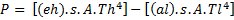(where the h refers to the high temperature emitting surface and l refers to the lower temperature absorbing surface, A is area).s is the Stefan-Boltzmann Constant = 5.6703 x 10-8 W/m2K4. When dealing with a problem like the sun radiating to the earth for power calculations, note that there is only a section of the area of the sun that radiates to the earth, the radiation is received over a certain section of the earth and that a larger area of the earth radiates away from the earth.  Note also that this law above is valid for most situations, however the application to structures where features have a less than the Wein wavelength -see below- could involve some correction terms.  Typically when in the room temperature range this is correction is for below ten micrometers and when at the MHI high temperature device range this is ~800nm.

The spectrum of radiation in the electromagnetic spectrum, is described in terms of its constituent frequency or wavelength of the radiation.   The total radiation emitted by a surface at a given temperature encompasses several wavelengths.  The temperature (T), and peak wavelength (λ) and the frequency (v) relationship is shown below.   Note that the visible spectrum region is only a small portion of the entire em spectrum.   Radiation from a body comprises of multiple frequencies that may be considered to sum to a form of a resultant wave.  What is commonly known as heat radiation lies in the Infrared part (longer than light wavelengths).  Ultraviolet and X-Ray radiation are much higher frequency radiation (shorter wavelengths).  These are the high energy radiation and penetrate matter more than infrared radiation.   Note that the radiative power follows a power law that is T^4.   Free radiating elements like Microheaters™, GlowPanels™, Thermoplates™ and Magnacoils™ are designed for maximizing the heat power output,   Furnaces on the other hand are optimized and designed for maximum temperature and low power loss (insulated).  When the transfer mechanism is convective the best power transfer is achieved by Airtorch.  When an extremely rapid power transfer is required, a new breakthrough device called the the Cascade e-ion may be employed.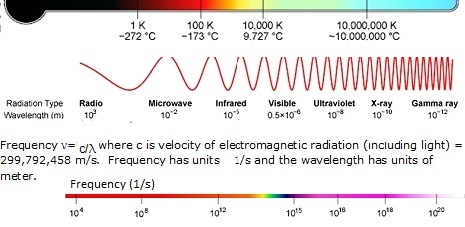Radiation can also be considered as comprising of photons with a spectrum of energies (this is the wave and particle duality).  A photon is a fundamental particle which represents a quantum of electromagnetic radiation. The energy of an individual photon is higher for photons of a higher frequency.  It is only the particles that are bound in potentials that get quantized energies. Thus the photons that mediate transitions between these quantized energy states are quantized.  This explains why even though the Planck radiation equation is expressed as a continuous variable of wavelength, one notes that the emission and absorption spectra of atoms only yield discrete line-signatures.  From solar cells to concrete testing to metal surface hardening, MHI devices utilize these differences in free electrons and bound electrons for useful device manipulation for specific applications.

The relationship between the energy and frequency is given by Planck's energy law for a Photon equation E = , where E is the energy per photon, ν is the frequency of the photon, and h is Planck's constant equal to 6.626×10−34 J.s.  When considering the wave-particle duality, the frequency of a wave is its rate of oscillation and is measured in Hertz, the SI unit of frequency, where one Hertz is equal to one oscillation per second.  A photon carries energy that is proportional to the 'frequency' of the radiation (or inversely with the wavelength as the velocity is constant).   Photons belong to a  class of fundamental particles called bosons. Photons are the force carriers of the electromagnetic field.   Photons are not conserved.  Photons have a zero rest (invariant) mass.  The number of photons per second is related inversely to the electromagnetic/ light frequency (probability).   The photons/second = Pλ/hc = P/hν where P is the Power in Watts, c is the speed of light, h is Planck's constant, and ν is the frequency.  The energy E of a photon is given in electron volts.  Red light photons are about 1.7 eV and blue light photons about 3.2eV (alternately we could have said that the red light wavelength is about 0.8 micron and blue light is 0.3 microns).  Note that  1eV=1.60218e-19 Joules. Note that the energy of a photon in eV (electron volts) is equal to 1.239/ (Wavelength in microns).

The radiation power density as a function of wavelength and temperature is given by the Planck's Radiation Law for Black Bodies (shown in the plot(s) below) - also called Planck formula.  S the represents Radiation Power Density, W/m3 per steridian or Spectral Irradiance per steradian obtained from Planck's  Radiation Law, a function of the wavelength.  Definition of steridian:  An area on a sphere which is equal in area to the square of its radius, when observed from its center, is precisely one steradian.  This is similar to the relationship between arc length and the angle in radians in two dimensions.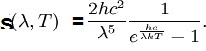The radiated power density (S) as a function of wavelength is shown below for different temperatures.The Planck's constant h = 6.626×10−34 joule-second (J.s)and c is the velocity of  electromagnetic radiation. Note that the power density increases with the temperature for any given wavelength. A peak (denoted as λmax) is also noted in the S vs wavelength plot for any given temperature .  This peak shifts to a lower wavelength (higher frequency) with an increase in the temperature. The peak wavelength and temperature are related by the Wein's radiation constant.  The mathematical relationship is λmax. T = W, where W is a radiation constant, λmax is the peak wavelength and T is the absolute temperature.  The W  is called the Wien's displacement constant equal to 2.8985×10−3 m·K.  The Planck equation (shown alongside the plots below) can be integrated over all wavelengths to yield the Stefan-Boltzmann law discussed above.  The units for the three graphs below should not to be confused with the units of P (the total power given by the Stefan Boltzmann law). the radiation equation was originally derived in a semi-emperical manner prior to the quantum/statistical methods discussed further below.

The power density S can also be derived by considering the radiation as comprisiong of photons.  The Planck's constant value 6.626176 x 10-34 J.s when multiplied by frequency is the smallest quanta of energy that can be carried by a corresponding photon. From a knowledge of the gravitational constant, the Planck Energy based on the Planck Mass is Mpc2 equal to 1.9561x 10^9 J.  Similarly a Planck's length ~1.6 x 10^-35 m (the distance travelled by radiation during one Planck-time is one Planck-length) based on Planck's time. ~5.39 x 10-44 seconds. These are the smallest scale(s) in the universe (because of the Uncertainity Principle), however, the numbers are too small to measure directly or verify for now. Although not directly related to the uncertainity principle, Gödel's incompleteness theorems on the limitations of possible knowledge of formal systems should also be studied by those interested.

The Planck's radiation formula for photons is an example of the distribution of energy predicted by Bose-Einstein statistics reflecting that the particles (photons) are indistinguishable and have integer spin. Two particles can be considered to be distinguishable if their separation is large compared to their DeBroglie wavelength (λ=h/p where p is the momentum which for a massless photon is E/c).  For air-gas molecules at STP (standard temperature and pressure), the molecules have a separation on the order of 3 nm and DeBroglie wavelengths of the order of ~ 0.03nm, which is much smaller.  The kinetic energy is thus spread in a manner that relects a Guassian type distribution of the kinetic energies of the gas atoms.  The mean energy in a distribution is the measure of temperature.  On the other hand, electrons and photons are indistinguishable particles but because of their quantum spin-numbers may follow different energy distribution statistics, namely Fermi-Dirac statistics for electrons and Bose-Einstein statistics for photons.  For completeness, we should mention here that lattice vibrations (phonons) are also indistinguishable particles but may follow different statistics at different temperatures. At extremely low temperatures, even the larger particles (atoms) can have have longer deBroglie wavelengths (i.e. as as p (the momentum) tends to zero) and so especially when the atoms are highly concentrated and at a very low temperature they can be considered as indistinguishable particles and 'condense' into what is called zero-friction superfluids. One should note that the "the standard model" that describes the make-up of all matter with numerous fundamental particles (even inside protons, neutrons and other constituents) is now able to integrate almost all interactions of particle intensity probabilities - however, following  Planck's law is OK for us for making thermal descriptions.  It does not matter which particles are being studied - nucleons or electrons- the quantum mechanical calculations are able to good progress within the Heisenberg Uncertainty Principle for making good predictions of forces and probability-polarity.  Regardless, experimental diffraction at very small length scales are not yet available for proper validation of these concepts.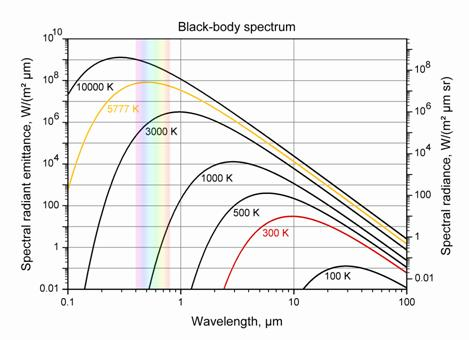In the visible region the range of photon energy with color is shown below.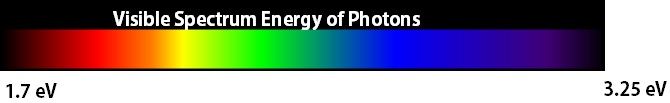These graphs are reproduced from the open source "Physics".   Note that the energy of a photon in eV (electron volts) is equal to 1.239/ (Wavelength in microns).

Radiation interacts with chemical bonds.  In the infrared region the vibrational states are affected as shown below.  In the visible and UV regions ionic dissociations (excited states) are feasible.

Sun/Greenhouse effect:  The sun is a black-body.  The greenhouse effect pertains to the earth receiving radiative energy with the distribution of wavelengths in the solar spectrum (from the sun which has a surface temperature of ~5778K), but then emitting and reflecting a spectrum associated with the much lower temperature of earth (~ 300K).  The emission is mostly in the IR (infrared wavelengths).  Molecules absorb characteristic wavelengths.  Some of the emitted and reflected (polarized) radiation from earth falls in the absorption band-characteristics of CO2, water vapor, fluorocarbon, and other gasses in air, thus trapping a considerable part of the emitted and reflected IR radiation (heat). The interaction whether elastic type or inelastic type influences reflection and radiation. The sun may be considered to be a black-body (e=1) while the earth may be considered to be a grey-body (with e=0.8) for making of any approximate calculations pertaining to the energy distribution. Note that only a grey body can reflect. It should be noted that the emissivity of the integrated emissivity of an object is a function of temperature.  For approximate energy calculations, it may inferred that the Sun radiates 6.3 x 10^7 W/m2.  The earth (over the atmospheric area of the planet radius) receives ~1.3 x 10^3 W/m2 sunlight and radiates (from its actual surface) ~(250-350) W/m2, which is considerably absorbed by gasses in the atmosphere. The units of P in the equations above for power transfer between objects at two temperatures are W or W/m2, from the Stefan-Boltzmann law.  The units of power density above S are W/m3 or W/m3 per steradian (y axis of the the graphs above) i.e. from Planck's Radiation Law formulation above.  The conversion between P and S at equilibrium involves the Wein's radiation constant or integration over the spectrum (all wavelengths) as described by Planck's Law for the Energy of a Photon namely that E = , where E is the energy per photon, ν is the frequency of the photon, and h is Planck's constant equal to 6.626×10−34 J.s.

Shown below is the color spectrum of visible radiation and the energy associated with a particular wavelength of light. The solar spectrum credit is to https://en.wikipedia.org/wiki/File:Solar_Spectrum.png.  Note that absorption spectra for O2, O3 and H2O are captured in the center graph but it does not call out methane absorption which is minor constituent (because of low atmospheric concentration, 20-40 ppm level) but important when methane gas leaks are encountered (methane and other such gas lines (bands) absorb in the 1.62, 3.2 and 8.8-9.2 micron range).

Methane is a much smaller component than carbon dioxide but a more potent driver of the greenhouse effect.  Natural gas has considerable methane content as does gas from biogenic sources. One other reason for the warming comes from the fact that ice (a solid) reflects a much larger fraction of sunlight than water which reflects only ~ 6% of the incident solar radiation. Technically speaking this happens because of  the extinction coefficient and the refractive index differences between a liquid and solid.  Sea-ice is thus important to prevent the warming because it reflects sunlight (50-70%), thereby reducing the amount of energy absorbed at the surface. The change in the amount absorbed when there is less ice formation, changes the pattern of water-currents/circulation.  An interesting observation is that snow (a semi-solid) can reflect ~90% of the incident solar radiation - even more than ice!. This is related to surface roughness and correlation length differences when comparing snow and ice surfaces.  Any surface can be optimized for emission and reflection in order to improve the energy efficiency of the objective.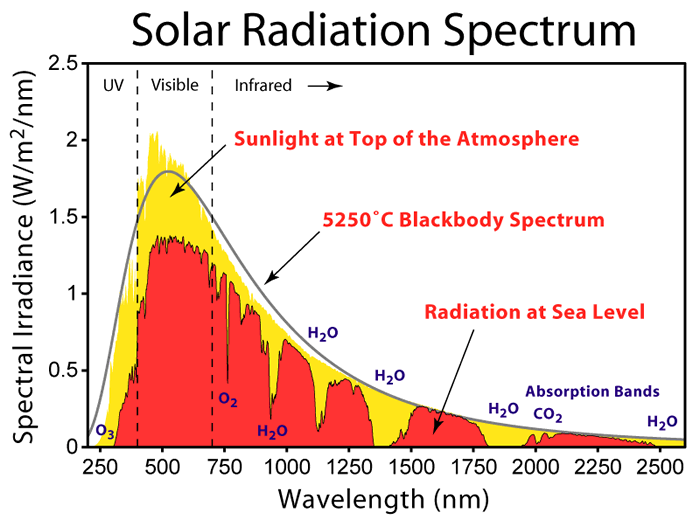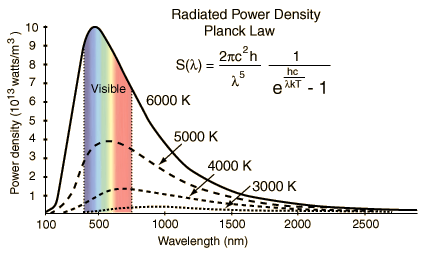The units of P in the equations above for power transfer between objects at two temperatures are W or W/m2, from the Stefan-Boltzmann law.  The units of power density above S are W/m3 per steradian (y axis of the the two graphs above) i.e. from Planck's Law.  The conversion between P and S at equilibrium involves the Wein's radiation constant or integration over the spectrum (all wavelengths) as described by Plancks Law.    The λmax = hc/zkTwhere z = 4.96511 (integration constant), which is Wein's law.   Here h is the Planck's constant and k is the Boltzmann constant. Note also that pressure has units of J/m^3 and so this radiation law also describes radiation pressure as a function of temperature and length scale, i.e., can thus be thought to be analogous to an 'ideal' equation of state for radiation.

The interaction of radiation with matter is quantized.  The range of photon energies in IR spectrum has possibilities of interaction as shown below with the vibrational state of molecules.  The UV-Vis spectrum of energies i.e. the higher energy photons, can interact with more excited states of electrons in the atomic, molecular and conduction bands.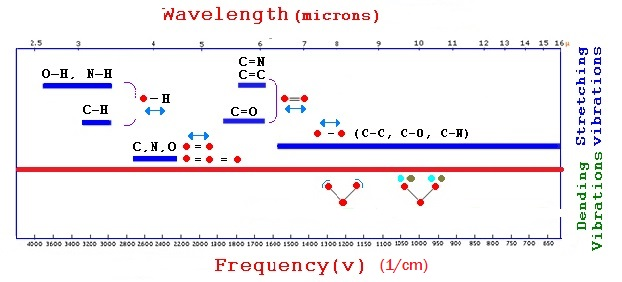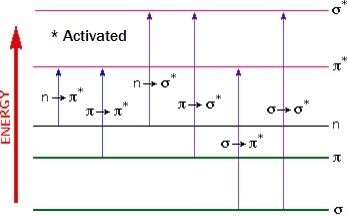IR Spectrum Devices

Somewhere in the range of 600C to 800C, the blackbody spectrum encroaches enough in the the visible to be seen as a dull red glow.  At temperatures in the range of 50oC, almost all of the radiated energy from the surface of a body or a wall lies in the infrared part of the spectrum (see above). The wavelengths in this part of the spectrum are of the order of (1000nm = 1 micron) and above. At low temperatures, the peak shifts to higher wavelength and as shown above could be mostly off the visible scale below 600oC.  Thus the human body mostly loses radiation (heat) by emitting in the infra-red (IR) region, invisible to the eye.   A typical human body controls its body temperature with a natural automatic regulation process and attempts to keep t around 37°C.   An average human-body emits about 100W of radiation at 37oC.  This number increases with vigorous exercise.

Detecting human body movement requires sensors that are particularly sensitive in the IR regions.   Night vision goggle have this type of feature that accentuates IR and converts it to a more visible frequency which the eye can detect.  Almost all surface heating involves IR. Each quanta of IR has a lower frequency compared to UV.  As the frequency increases towards UV radiation the waves penetrate more as they have more energy.  The energy of a photon depends on the wavelength.  This is why UV shades are recommended  because UV has a shorter wavelength than IR.  Note that the radiation power of a surface increases with temperature and with the emissivity in a non-linear manner.  Note also the general trend of emissivity of a materials is to increase as a function of temperature.  However emissivity and reflectance are not simple properties.  Radiation is absorbed via mechanisms that involve allowed quantum states.  The dielectric constant has a real and imaginary component and directly impacts reflectance (mostly from the real part). However the refractive index and texture caused dispersion is noted in reflectance measurements e.g. reflectance for a shiny metal can often decreases with peak temperature or increase because of plasmonic influence.  Emissivity is associated with high temperature measurements (>300K), reflectance with low (<300K) temperature measurements.

Visible Spectrum Devices

Solar and UV panels can be of two types (1) those that absorb all radiation (black body) in the solar spectrum, generally used for heating  and (2) that selectively absorb in order to stimulate electron activity in semiconductors.  These are classified as visible spectrum devices.  The cascade e-ion devices may be used to produce a combination of the two types,  The cascade e-ion is a device that is able to make oxynitride, carbonitride, nitride and carbide surfaces/coatings of transition metals.Surfaces can be manipulated to absorb some wavelengths and reflect the others. Such surfaces made by Cascade e-ion or D-e-ion devices also often impart antimicrobial properties.   MHI's patented high emissivity and controlled boson, fermion, photon stimulators are the key to the next generation of power producing surfaces.  See also deburr page for Golden/Blue surfaces/ microstructures for iron, zirconium and titanium alloys.   As noted above, Kirchoffs law applies, however the amount transmitted or reflected at various wavelengths can be manipulated with transparent coatings e.g. of glass or gold plating or the more recently rediscovered copper-tin mirror materials.  Variations is the surfaces made by the cascade e-ion are are being discovered e.g. a black body in the visible spectrum range can be covered with a layer (glass) which is transparent in the visible range but opaque in the UV or IR range. Such layering techniques may be used for example for hot water solar heaters.

How is light measured? What do Lumens and Lux signify?  What is Brilliance?

Brilliance in the context of light, is related to the photons per second that our eye senses.  In the visible spectrum, the units of lumens (amount of light in the visible range) and lux are used to calculate/infer the illumination which normally pertains only to the visible spectrum objectives. Lumens is calculated by knowing the spectrum or number of photons per second in the "light" frequencies and converting it to an absolute number in a slightly round about manner.  The efficiency of the device for the objective of providing illumination is defined by the luminous efficacy η in lumens per watt of the device (lm/W).  The illuminance Ev in lux (lx) is equal to the power P in watts (W), multiplied the luminous efficacy η, divided by the surface area A (m2):  Ev(lx) = P(W) × η(lm/W) / A(m2) .   Note that red is a higher wavelength radiation compared to violet.  In terms of wavelength, the graphics above and below for the energy of photon (shown in eV) would range from 720 microns to 390 microns (left to right). The power of radiation given in photons per second and the lumens are related to brightness by number of photon per second in the visible range.

Luminous intensity should not be confused with luminous flux, which is the total perceived power emitted in all directions. Luminous intensity is the perceived power per unit solid angle. If a lamp has a 1 lumen bulb and the optics of the lamp are set up to focus the light evenly into a 1 steridian beam, then the beam would have a luminous intensity of 1 candela.  The candela is the luminous intensity, in a given direction, of a source that emits monochromatic radiation of frequency 540×1012 hertz (green) and that has a radiant intensity in that direction of 1/683 watt per steradian.

Different light emitters can now be compared - LED source gives 90 lumens per Watt  compared to a incandescent bulb which is about 30 lumens per Watt. The best estimate is that theoretically one watt of power in the ~540 nm wavelenght range can at best result in ~680 lumens (lm).   The good news is that every year appears to bring a cost reduction with an increase in lumens/watt/device - a twenty year trend that is good.

Lux as described above is a measure of the flux of lumens per unit area.  Remember we have used the word brightness above a bit loosely as it depends on the eye and other aspects of roughness and color i.e. on the number of photons per second that we can observe.

A few rules of reflection intensity

Although the main law of reflection states that the angle of incidence and reflection are equal, one can understand that the texture and waviness that is always present on a real surface will alter the local angle of incidence across a beam of incident radiation. Smooth surfaces that behave like a mirror are referred to as specular reflectors. Otherwise they form certain classes of diffuse reflectors.  A rough surface is defined as having a surface texture height variation that is greater than half the incident wavelength (λ).  Surface roughness is defined with respect to wavelength and incidence angle.  Surfaces will appear to have a degree of roughness depending on which wavelength is imaged. When imaged, a surface will appear to be smooth if its average height variation is smaller than one-eighth of the wavelength. Therefore IR radiation (~1um) will be reflected more predictably by ~100nm rough surfaces (the reflected amount depends also on the complex refractive index and absorption characteristics of the material).  However the same surface may appear rough and less predictable for the intensity of reflection for imaging by blue light.  Or within a visible spectrum examination, a surface will seem less rough in red light than when seen in green light.  A surface will also appear to be smooth as the incidence angle increases.  New results are begining to indicate that scattering by nanofeatures happens in a strange manner that increases the reflectivity of smaller wavelenghths of light as the features become smaller in size thus producing unanticipated colors.  If light is scattered back in the direction of incidence by a rough surface, it is referred to as backscattered light.  Many sensors are based on backscattered light, so for many images rougher surfaces will appear brighter because of such sensors.

The color of a surface?  Thin Film Interference.

The reflection color from an object for sunlight depends on the intensity (including angle), polarization, photonic band gap, refractive index (real and imaginary parts) and other properties usch as roughness and multiscale nano periodicity (see previous paragraph). The reflection or refraction of individual wavelength may not be the same for all surfaces or thin films. Color separation often occurs just like in a prism but for different reasons. Butterfly wings have tiny scales covered with microscopic ridges and other structures. These play with light waves to create brilliant purple/blues and speckles of colors. The structure, instead of a colorong chemical, creates the concentration of wavelenghts (color). They may also describe nano-scale texture. Such separation properties are also utilized for thin film devices. Thin film interference is a phenomena associated with films which determines colors. Thin films have many commercial applications including as partially transparent or anti-reflection coatings, mirrors, and on optical filters.  For graded and compositionally variant films, the colors yield considerable important information about a coating or thin layer. The thin film interference phenomena partially explains the colors of oxynitrided and nitrideded surfaces, tempered colors, and also the color of the wings of butterflies!  The reflected, partially reflected, or refracted wave (depending on polarization) may interact and thus reveal information about the surface(s) from which individual frequency components are reflected, e.g. information about the thickness of the film, or the effective refractive index of the film medium.  The refelection intensity is dependent on the refractive index,  extinction coefficient and surface texture.Span of Visible Photon Energy with Color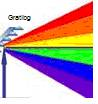Color Grating

Various colors of oxynitrides are often noted on a steel surface a shown below.    These are called colors of tempering.  Amzingly these colors are seen also in cobalt and aluminum alloys and are not just for steels.    The color varies with the type of metal, time of film formation, phases, atmosphere/thickness and incident light distribution and other surface textures.  Note specular and matte finishes below are both possible for the common variations that are noted on iron and steel surfaces.

Why do these colors form on nano-surfaces?   From thin film interference and from back-scattered as well as diffuse-reflectance variations.

A surface appears smooth to the eye, when the roughness is about a half to eights of the measurement wavelenght i.e. less than 500 nm (mid point) for visible radiation) and when viewed at a high angle of incidence. Such surfaces can appear to be colored bluish when the auto-correlation length is small as well as the mean asperity roughnesses is very small at these texture levels although effects of the surface constituents (phases) as described below are also dominant. The colors of titanium, zirconium and alloy oxynitrides vary from red-yellow to blue/violet indicating also some variations in the band gap of the material that are enabled by changes in the the oxygen/nitrogen ratio.  Texture compositing can create many colors when different phases coexist at a surface.  Thin film interference, absorption coefficients and internal scattering by small-scale phases like Fe2(CN) play a role in the color formation. There is some analogy with the prism light grating shown above, however the closest scientific analogy to the tempering colors of oxides and nitrides is noted in water-droplet residual drying colors on a surface, the colorful wings of an attractive butterfly (morpho-color), the spectacular color separation obtained from a prism-light grating, the red-shift explanation from moving objects, and the commonly observed variations in the color of the sky and across moonbows and rainbows.  However there are very few clear explanations that comprehensively explain all oxynitride surface colors.  Reflection, polarization and a complex refrative index with a real part close to 1.55 seems important but there is much more to be learnt about multi-scale roughnesses.